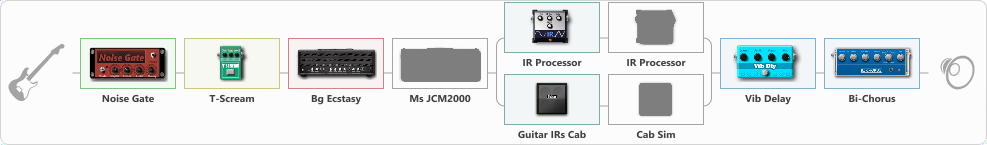# Carlos-2

Discussion in 'ToneLib-GFX presets' started by carlinos3, May 11, 2020.

1. Carlos-2

Preset name: carlos-2

Effects chain:Effect: "Noise Gate" (Dynamics / Filter), active - "yes"
{
"Mode" = Auto
"Depth" = 50
"Threshold" = 50
"Attack" = 0
"Hold" = 5
"Decay" = 250
}

Effect: "T-Scream" (Overdrive / Distortion), active - "yes"
{
"Drive" = 22
"Tone" = 80
"Level" = 79
}

Effect: "Bg Ecstasy" (Amp simulators), active - "yes"
{
"Gain" = 100
"Bass" = 50
"Middle" = 50
"Treble" = 75
"Presence" = 100
"Master" = 75
"Level (dB)" = 2
}

Effect: "Ms JCM2000" (Amp simulators), active - "no"
{
"Gain" = 100
"Bass" = 50
"Middle" = 50
"Treble" = 50
"Presence" = 80
"Master" = 69
"Level (dB)" = 5
}

Effect: "Splitter" (Dynamics / Filter), active - "yes"
{
"A-Bypass" = Off
"A-Pan" = 1
"A-Level" = 62
"B-Bypass" = Off
"B-Pan" = 1
"B-Level" = 32

'A' branch:
{

Effect: "IR Processor" (Cabinets), active - "yes"
{
"IR" = charv.1_dc
"Low Cut (Hz)" = 0
"Hi Cut (kHz)" = 20.0
"Mix" = 100
"Level (dB)" = 4
}

Effect: "IR Processor" (Cabinets), active - "no"
{
"IR" = 340_dc
"Low Cut (Hz)" = 0
"Hi Cut (kHz)" = 20.0
"Mix" = 100
"Level (dB)" = 5
}
}
'B' branch:
{

Effect: "Guitar IRs Cab" (Cabinets), active - "yes"
{
"Model" = Bogner Uberkab (4x12")
"Mic Position" = Center
"Mic Distance" = Near
"Low Cut (Hz)" = 60
"Hi Cut (kHz)" = 20.0
"Mix" = 100
"Level (dB)" = 5
}

Effect: "Cab Sim" (Cabinets), active - "no"
{
"Model" = 1x8" Small Tweed
"Level (dB)" = 5
}
}
}

Effect: "Vib Delay" (Modulation / Sfx), active - "yes"
{
"Speed" = 4.2
"Depth" = 38
"Feedback" = 28
"Time" = 5.7
"Mix" = 37
"Mode" = 0
}

Effect: "Bi-Chorus" (Modulation / Sfx), active - "yes"
{
"Speed A" = 3.2
"Speed B" = 4.0
"Depth" = 43
"Reso" = 0
"Mode" = Parallel
"Mix" = 14
}

Note: You will need to download and install the ToneLib-GFX software to use the preset.

#### Attached Files:

• ###### Carlos-2.tlgfx
File size:
127 KB
Views:
2,737
York, sonar72 and no-name like this.